# Question 23 Find the maximum of f(x, y) = 5x 3y subject to the constraint x?...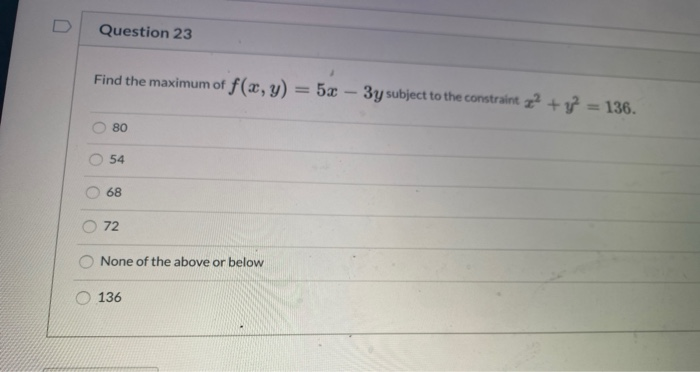Question 23 Find the maximum of f(x, y) = 5x 3y subject to the constraint x? + y =136. 80 54 68 72 None of the above or below 136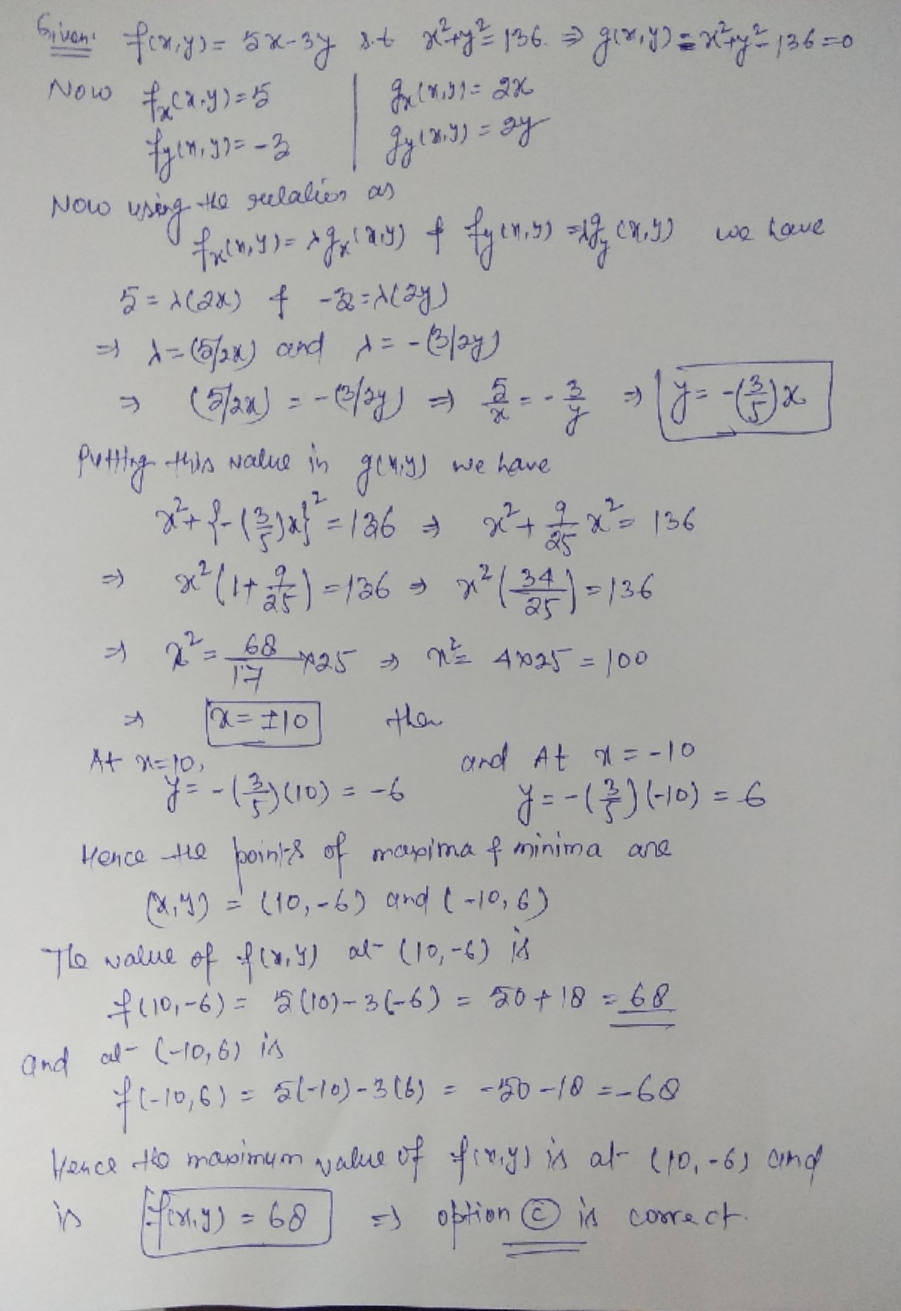##### Add Answer of: Question 23 Find the maximum of f(x, y) = 5x 3y subject to the constraint x?...
Similar Homework Help Questions
• ### 3 Find the minimum and maximum values of the function f(x, y)= x +y subject to the constraint x + y 1250. Use the L...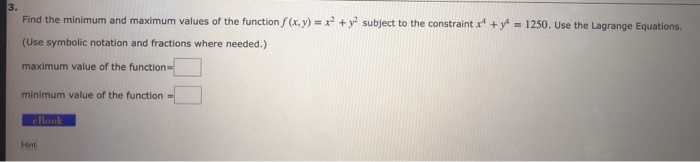3 Find the minimum and maximum values of the function f(x, y)= x +y subject to the constraint x + y 1250. Use the Lagrange Equations. (Use symbolic notation and fractions where needed.) maximum value of the function minimum value of the function cBook Hint 3 Find the minimum and maximum values of the function f(x, y)= x +y subject to the constraint x + y 1250. Use the Lagrange Equations. (Use symbolic notation and fractions where needed.) maximum value...

• ### 3. Find the minimum and maximum values of the function f (x, y) = x2 + y subject to the constraint x y = 162. Use the L...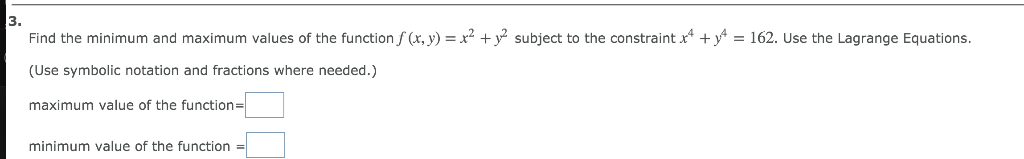3. Find the minimum and maximum values of the function f (x, y) = x2 + y subject to the constraint x y = 162. Use the Lagrange Equations. (Use symbolic notation and fractions where needed.) maximum value of the function| minimum value of the function 3. Find the minimum and maximum values of the function f (x, y) = x2 + y subject to the constraint x y = 162. Use the Lagrange Equations. (Use symbolic notation and fractions...

• ### 9. Find the maximum and minimum of f(x,y) = 4.r+10y2 subject to the constraint x2 +...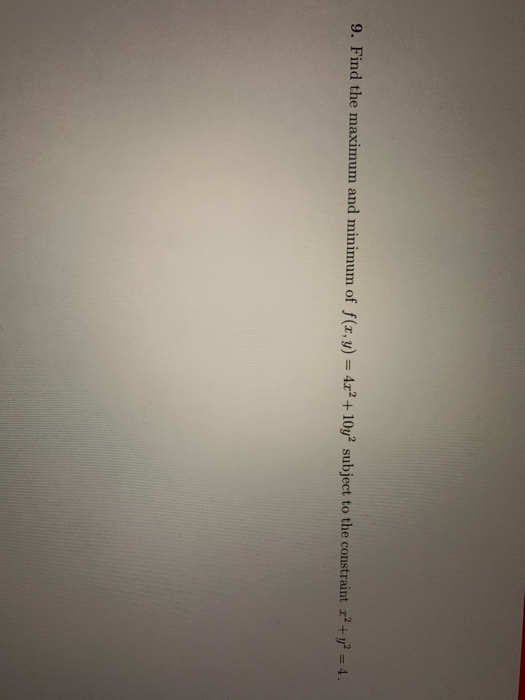9. Find the maximum and minimum of f(x,y) = 4.r+10y2 subject to the constraint x2 + y2 = 4.

• ### Find the minimum and maximum values of the function subject to the given constraint.

Find the minimum and maximum values of the function subject to the given constraint.f(x, y, z)=x2 - y - z,x2 - y2 + z= 0

• ### Find the maximum and minimum values of the function f(x,y,z)=x^2y^2z^2 subject to the constraint...

Find the maximum and minimum values of the function f(x,y,z)=x^2y^2z^2 subject to the constraint x^2 + y^2+ z^2=36. The maximum value is _______________ occuringat________________ points (positive integer or "infinitely many"). Minimum value is ________________ occuring at ________________ points (positive integer or "infinitelymany").I found Max to be 12^3 though i cant find anything else

• ### Use Lagrange multipliers to find the maximum and minimum values of the function subject to the given constraint. (If a v...

Use Lagrange multipliers to find the maximum and minimum values of the function subject to the given constraint. (If a value does not exist, enter NONE.) f(x,y,z) = x2 + y2 + z2;   x4 + y4 + z4 = 1

• ### 28.- Use Lagrange Multipliers to find the maximum and minimum values of f subject to the given constraint 4x2 +8y2...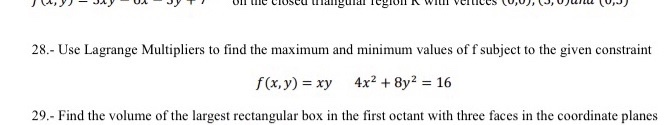28.- Use Lagrange Multipliers to find the maximum and minimum values of f subject to the given constraint 4x2 +8y2 16 f(x,y) -xy 29.- Find the volume of the largest rectangular box in the first octant with three faces in the coordinate planes 28.- Use Lagrange Multipliers to find the maximum and minimum values of f subject to the given constraint 4x2 +8y2 16 f(x,y) -xy 29.- Find the volume of the largest rectangular box in the first octant with...

• ### Find the maximum and minimum values of the function subject to the constraint

f(x,y,z)=x^2y^2z^2 subject to the constraint x^2+y^2+z^2=324Maximum value is ? occur at ?Minimum value is ? occur at ?NOTE: please I want a full work

• ### Follow the instruction below to find the maximum value ofz = 5x -2ysubject to the constraintsx+y;::22x + 3y S 12 3x + 2y S 12 x;:: 0, y;::Oa) Graph the feasible region

2. Follow the instruction below to find the maximum value ofz = 5x -2ysubject to the constraintsx+y;::22x + 3y S 12 3x + 2y S 12 x;:: 0, y;::Oa) Graph the feasible region.b) Find the comers of the feasible region. c) Find the maximum of the function z.

• ### Use Lagrange multipliers to find the ends of f(x,y)= 2x2 +3y2 subject to the constraint 3x...

Use Lagrange multipliers to find the ends of f(x,y)= 2x2 +3y2 subject to the constraint 3x + 4y = 59

Free Homework App